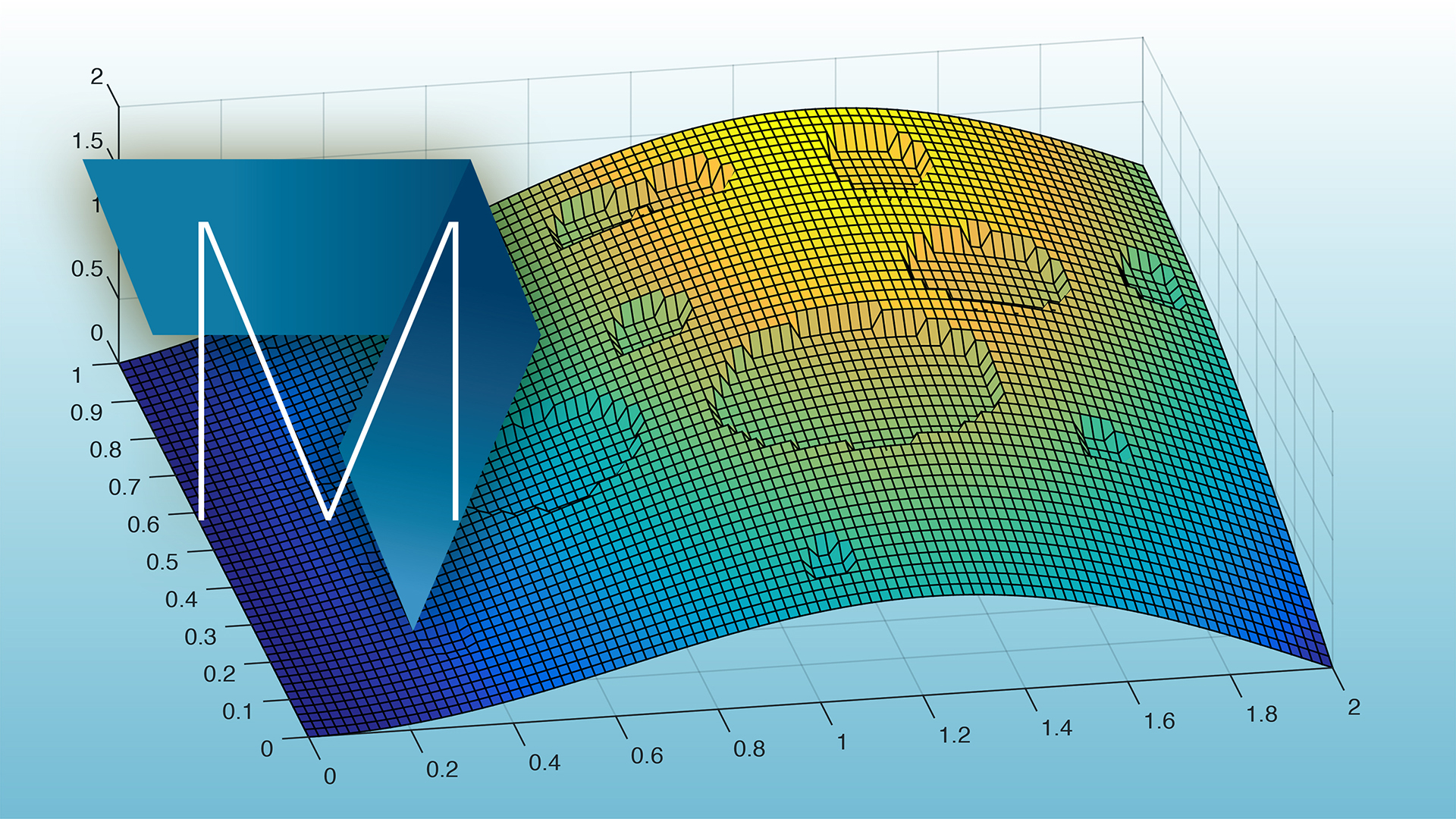# MATLAB and Octave for beginners

EPFL### MATLAB and Octave for beginners

The course you are about to follow is an introduction to à Octave and MATLAB®. Both of these softwares follow the same structure, it is therefore obvious to present them together. This course is aimed at beginners, it began as an introduction to MATLAB for second year students at EPFL following a numerical analysis course.

### What will you learn

• Work with vectors and matrices
• Process text files containing data
• Manipulation of plots and figures and saving them to pdf or jpg
• Using scripts and functions
• Writing of small interactive programs

Simone Deparis

### Other MOOCs from EPFL

You can find here more information on the MOOCs given by l'EPFL. The following courses could be of interest for you:

Linear Algebra

Preparation course: Trigonometric, Logarithmic and Exponential Functions### How to move through this course

Start the course by clicking the below menu. An introduction will guide you through the course.

If this is your first course on the edX platform, we recommend that you follow the demo course on edX before the course on Octave and MATLAB starts. This course presents the platform, shows you how to answer questions and follow your progress.

1. Course Number

MatlabOctaveBeginners
2. Classes Start

Enroll